#### Tech Note

Michael Faraday in 1831 and Joseph Henry in 1832 independently discovered electromagnetism.(3) Faraday published first and gets the honor of his name on the phenomenon.

Faraday’s law of induction is a basic law of electromagnetism. When an electric circuit is moved in the neighborhood of a magnet, a current is induced in the circuit during the movement. Interestingly, it does not matter whether the circuit moves or the magnetic moves. There will also be a resistive force experienced. This is how electric motors and generators work.

Current in a wire also causes a magnetic field to exist in the neighborhood of the wire. A permanent magnet or a current carrying wire both represent electrons in motion, non-random motion. This field may be concentrated by a high permittivity, iron or ferrite material. Transformers represent one of the most common examples of Faraday’s Law.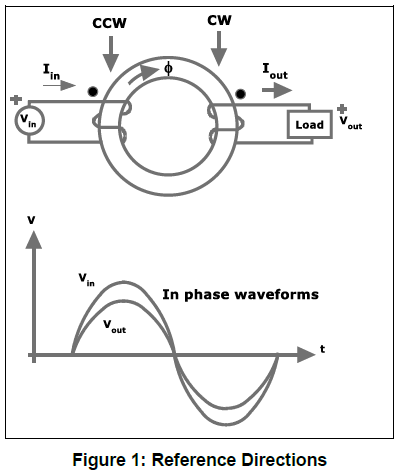Figure 1 represents an experimental result using a toroid transformer. The input current causes a magnetic field in a ferrite or iron toroid. The direction of this field Ø is given by the convention of the right hand rule. Thumb in the direction of current and fingers will be in the direction of the field. Why does go in the direction shown? When energy is stored in the magnetic field its flux direction follows the right hand rule. When energy is released from the magnetic field, the right hand rule indicates the output current apparently producing flux opposing the input flux. This behavior is known as Lenz’s law.

Another explanation given by some writers is this. Consider the opposite, if it went the other way, would it not cause additional field flux adding to the original and causing even more current in the input circuit? That would be a violation of the conservation of energy and Newton’s third law, for every action there is an equal and opposite reaction.This phenomenon will be caused by moving a field source toward or away from a coil of wire, or moving a coil of wire into or out of the field. You can also rotate the coil in the magnetic field. The voltage generated in the coil is proportional to the negative of the rate of change of the field.

Faraday’s explanation talked of lines of force; however, other scientist at the time rejected his theory because it was not expressed mathematically. It was James Clerk Maxwell in 1862 who took this experimental phenomenon into his electromagnetic theory expressed in the famous Maxwell’s Equations. These four differential equations are the classical, mathematical basis of electricity and magnetism.

Faraday’s law stated mathematically is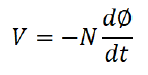N is the number of turns in a coil. Ø is the total flux passing through the coil. This simple form assumes the flux is perpendicular to a flat coil. A conductor parallel to magnetic flux has no emf excited. A flat coil whose plane is parallel to the flux has no net emf excited; since each incremental section has an opposing section on the other side. Flux has to pass through the loop to produce a net emf. A slightly more refined form of the law is,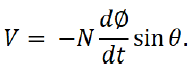𝛳 is the angle between Ø and a wire segment.

This is not exactly what Faraday described but was called Faraday’s Law by Oliver Heaviside. It does not include the movement emf; that is the force effect Faraday found. The magnetic force is called Lorentz force. Current flowing in a wire in the presence of a magnetic field will experience a force and move if not restrained. In this case, magnetic energy is released kinetically.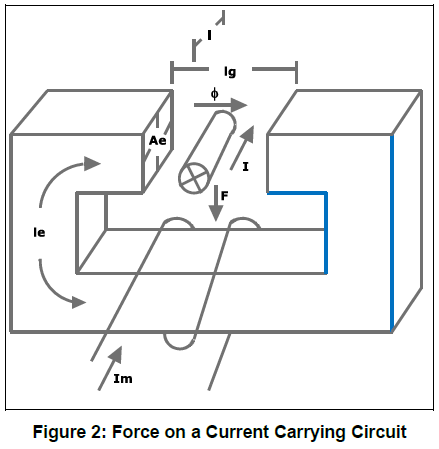A right-hand rule for force is fingers in the direction of current and rotate the palm in the direction of flux and the thumb will be in the direction of force.

The previous mention of the relative motion of magnetic field and electric circuit has had considerable thought by many, well-known physicists. Richard Feynman stated: (1)

So the "flux rule" that the emf in a circuit is equal to the rate of change of the magnetic flux through the circuit applies whether the flux changes because the field changes or because the circuit moves (or both) …

Yet in our explanation for the rule we have used two completely distinct laws for the two cases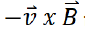for "circuit moves" and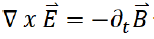for "field changes".

We know of no other place in physics where such a simple and accurate general principle requires for its real understanding an analysis in terms of two different phenomena.

Richard P. Feynman, The Feynman Lectures on Physics
Reflections on this duality may be one path that lead Einstein to develop special relativity.(2) The first paragraph of Einstein’s famous paper of 1905 discusses magnetism and electricity.
For if the magnet is in motion and the conductor at rest, there arises in the neighborhood of the magnet an electric field with a certain definite energy, producing a current at the places where parts of the conductor are situated. But if the magnet is stationary and the conductor in motion, no electric field arises in the neighborhood of the magnet.

Einstein then goes on to discuss the invariance of light speed. Of course, light is just another expression of electrodynamics. Additional treatment of electromotive forces is in section II of Einstein’s paper. This includes Doppler’s principle and the pressure of light on reflectors. Einstein starts with a set of postulates and works through to a resolution. The principle postulates are two: “the phenomena of electrodynamics as well as of mechanics possess no properties corresponding to the idea of absolute rest.” and “that light is always propagated in empty space with a definite velocity c which is independent of the state of motion of the emitting body.” The resolution stands the test of all known experiments. No stationary point is needed to explain electromagnetism and kinetics, hence the name “relativity.” Two or more English translations may be found by internet search. The 1923 translation is believed to have one or more symbol mistakes. There is also two uses of the same symbol causing possible confusion. See the footnotes of (2).

(1) "The flux rule" is the terminology that Feynman uses to refer to the law relating magnetic flux to EMF. Feynman, R. P. (2006). Leighton, R. B.; Sands, M. L., eds. The Feynman Lectures on Physics. San Francisco: Pearson/Addison-Wesley. Vol. II, p. 17-2. ISBN 0-8053-9049-9.

(2) On the Electrodynamics of Moving Bodies, A. Einstein, June 30, 1905 (http://www.fourmilab.ch/etexts/einstein/specrel/specrel.pdf) English Translation as published in the 1923 book The Principle of Relativity.